# 1. Whole Number on the number line:

A line that graphically represents the real numbers as a series of points whose distance from an origin is proportional to their value.

The distance between these points labelled as 0 and 1 is called unit distance.

A number written on the left-hand side of the number line is the smaller number and the number written on the right-hand side of the number line is the greater number.

We take a line, mark a point on it and label it 0. We then mark out points to the right of 0, at equal intervals. Label them as 1, 2, 3,... Thus, we have a number line with the whole numbers represented on it. We can easily perform the number operations of addition, subtraction, and multiplication on the number line.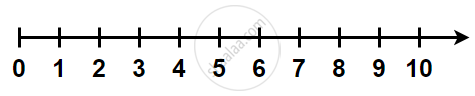# 2. Operation on Number Line:

### 1) Addition on the number line:

Let us see the addition of 2 and 5.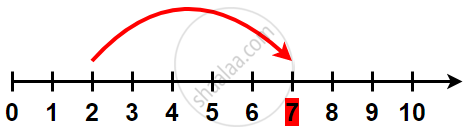Start from 2. Then move 5 places to the right will give 7. The sum of 2 and 5 is 7, i.e. 2 + 5 = 7. Addition corresponds to moving to the right on the number line.

### 2) Subtraction on the number line:

Let us find 9 – 6.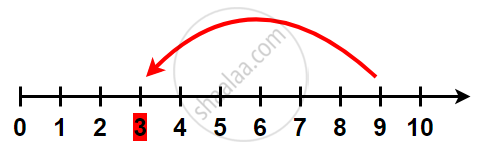Start from 9. Since 6 is being subtracted, so move 6 places towards left will give 3. We get 7 – 5 = 2. Subtraction corresponds to moving to the left on the number line.

### 3) Multiplication on the number line:

Multiplication corresponds to making jumps of equal distance starting from zero.

Let us find 2 × 6.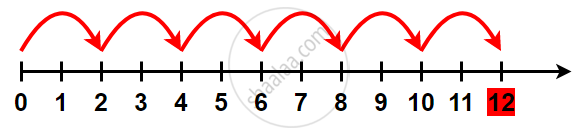Start from 0, move 2 units at a time to the right, make such 6 moves.

You will reach 12. So, we say, 2 × 6 = 12.

### 4) Division on a number line: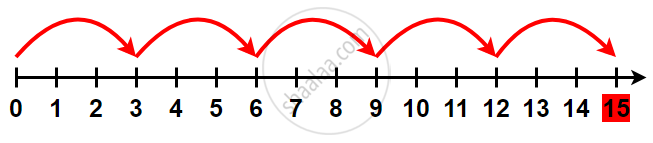A number line can be used to divide. To work out 15 ÷ 3, count how many 'jumps' of 3 it takes to get from 0 to 15. 5 'jumps' or 'groups' of 5 means 15 ÷ 3 = 5.

If you would like to contribute notes or other learning material, please submit them using the button below.

### Shaalaa.com

What is a Number Line? (CBSE 6th STD) [00:21:30]
S
0%